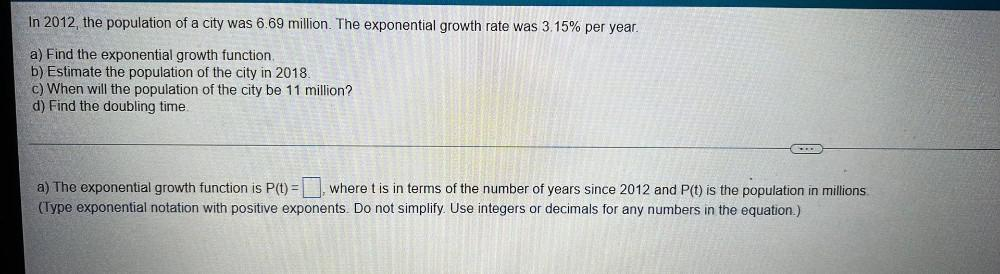Question:

# In 2012, the population of a city was 6.69 million. The exponential growth rate was 3.15% per year. a) Find the exponential growth function. b) Estimate the population of the city in 2018. c) When wilIn 2012, the population of a city was 6.69 million. The exponential growth rate was 3.15% per year. a) Find the exponential growth function. b) Estimate the population of the city in 2018. c) When will the population of the city be 11 million? d) Find the doubling time. a) The exponential growth function is P(t) = ▢ where t is in terms of the number of years since 2012 and P(t) is the population in millions. (Type exponential notation with positive exponents. Do not simplify. Use integers or decimals for any numbers in the equation.)